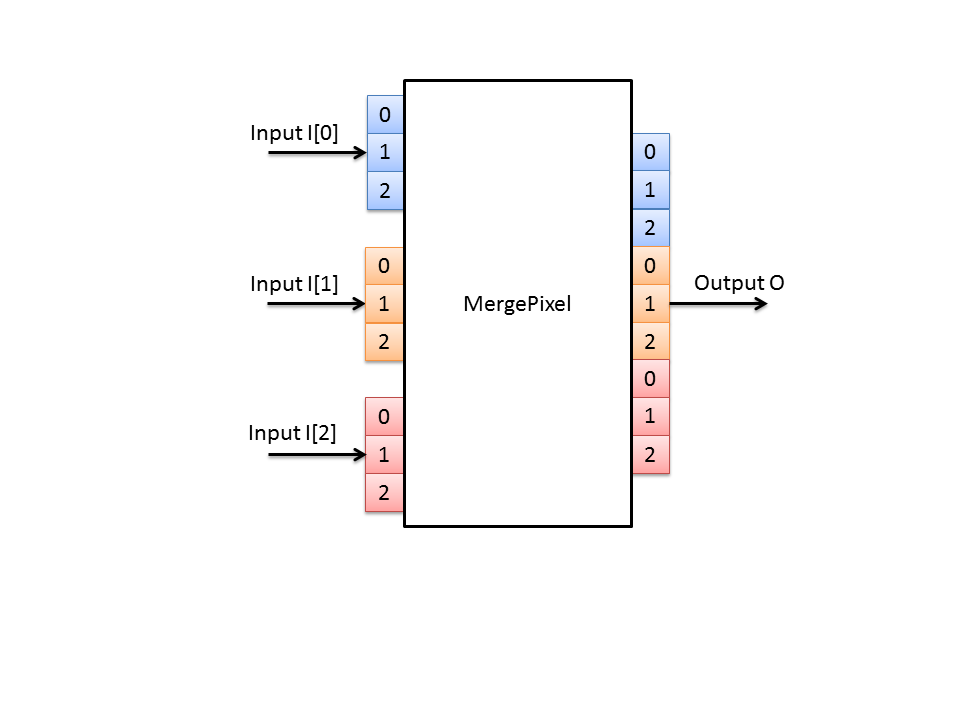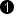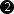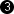## Operator MergePixel

The operator MergePixel merges pixels of multiple input links of the same parallelism into one pixel of larger bit width. The bits of the inputs are concatenated. The bits of input I0 will be mapped to the lower bits at the output. All inputs may have varying bit widths. The output is always unsigned. If signed pixels are merged, the bits are simply reinterpreted and concatenated.

Each color component is merged separately.

The following figure shows the merge of three input links into one link.### I/O Properties

Property Value
Operator Type O
I[n], n > 0, data input

Bit Width [1, 63][1, 63]autoArithmetic {unsigned, signed} {unsigned, signed} unsigned
Parallelism any as I0 as I0
Kernel Columns 1 as I0 as I0
Kernel Rows 1 as I0 as I0
Img Protocol {VALT_IMAGE2D, VALT_LINE1D, VALT_PIXEL0D} as I0 as I0
Color Format any as I0 as I0
Color Flavor any as I0 as I0
Max. Img Width any as I0 as I0
Max. Img Height any as I0 as I0
 The range of the input bit width is [1, 63] for unsigned values. For signed inputs, the range is [2, 63]. The sum of the input bits of all inputs must be <= 64. The output bit width is the sum of the bit widths of all inputs. The output bit width must be <= 64.

None

### Examples of Use

The use of operator MergePixel is shown in the following examples:

• 'RGB Camera Link Medium Area'

Tutorial - Basic Acquisition

• 'Grayscale Camera Link Full Area'

Tutorial - Basic Acquisition

• 'Laser Triangulation'

Examples - A high speed and robust laser line detection algorithm. The algorithm determines center of gravity coordinates to obtain sub-pixel resolution results.

• 'Simple Threshold Binarization'

Simple thresholding for binarization.

• 'Blob 1D'

Examples - Shows the usage of operator Blob_Analysis_1D in line scan applications.

• 'Blob 2D'

Examples - Shows the usage of operator Blob_Analysis_2D. The applet binarizes the input data and determines the blob analysis results. The results as well as the original image are output using two DMA channels.

• 'Motion Detection'

Examples - Calculates the differences between two successive images. The differences are thresholded and output via DMA channel.

• 'ED Morphological Edge Detection'

Examples - A binary eroded image is compared with the original. An edge is detected if both differ.